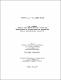## Topics in analytic number theory2016
##### Authors
In this thesis, we investigate three topics belonging to the probabilistic, classical and modern branches of analytic number theory. Our first result concerns the probabilistic distribution of squares modulo a composite number, and of tuples of reduced residues, in short intervals. We obtain variance upper bounds generalizing those of Montgomery and Vaughan, as well as new lower bounds. Our second work, joint with Nathan Ng, concerns the estimation of discrete mean values of Dirichlet polynomials, where summation is over the zeros of an $L$-function attached to an automorphic representation. Conditionally on strong bounds on autocorrelations of the coefficients of $L$-functions, a corollary of our results is that the gaps between consecutive zeros of the Riemann zeta function are infinitely often smaller than half of the average gap. Our last work concerns the additive and quadratic divisor problem. We study shifted convolution sums for the divisor function, Fourier coefficients of a cusp form and the representation function of integers as sums of two squares. For convolution sums of a certain type, we improve several estimates available in the literature, by expanding the delta-method of Duke, Friedlander and Iwaniec. Also by using a smooth variant of Dirichlet hyperbola method, we improve the error term obtained by Duke, Friedlander and Iwaniec in the quadratic divisor problem.